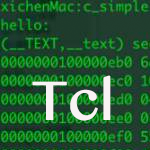# TCL运算符

• 算术运算符
• 关系运算符
• 逻辑运算符
• 位运算符
• 三元运算符

## 算术运算符

+ 两个操作数相加 A + B = 30
- 第一个操作数减去第二个操作数 A - B = -10
* 两个操作数相乘 A * B = 200
/ 除法分子通过去分母 B / A = 2
% 模运算及整数除法后的余数 B % A = 0

## 关系运算符

== 检查两个操作数的值是否相等，如果是的话那么条件为真。 (A == B) 不为 true.
!= 检查两个操作数的值是否相等，如果值不相等，则条件为真。 (A != B) 为 true.
> 检查左边的操作数的值是否大于右操作数的值，如果是的话那么条件为真。 (A > B) 不为  true.
< 检查左边的操作数的值是否小于右操作数的值，如果是的话那么条件为真。 (A < B) 为 true.
>= 检查左边的操作数的值是否大于或等于右操作数的值，如果是的话那么条件为真。 (A >= B) 不为 true.
<= 检查左边的操作数的值是否小于或等于右操作数的值，如果是的话那么条件为真。 (A <= B) 为 true.

## 逻辑运算符

&& 所谓逻辑与操作。如果两个操作数都非零，则条件变为真。 (A && B) 为 false.
|| 所谓的逻辑或操作。如果任何两个操作数是非零，则条件变为真。 (A || B) 为 true.
! 所谓逻辑非运算符。使用反转操作数的逻辑状态。如果条件为真，那么逻辑非运算符为假。 !(A && B) 为 true.

## 位运算符

p q p & q p | q p ^ q
0 0 0 0 0
0 1 0 1 1
1 1 1 1 0
1 0 0 1 1

A = 0011 1100

B = 0000 1101

-----------------

A&B = 0000 1100

A|B = 0011 1101

A^B = 0011 0001

& 二进制和操作符副本位的结果，如果它存在于两个操作数。 (A & B) = 12, 也就是 0000 1100
| 二进制或操作拷贝位，如果它存在一个操作数中。 (A | B) = 61, 也就是 0011 1101
^ 二进制异或操作符的副本，如果它被设置在一个操作数而不是两个比特。 (A ^ B) = 49, 也就是 0011 0001
<< 二进制左移位运算符。左边的操作数的值向左移动由右操作数指定的位数。 A << 2 = 240 也就是 1111 0000
>> 二进制向右移位运算符。左边的操作数的值由右操作数指定的位数向右移动。 A >> 2 = 15 也就是 0000 1111

## 三元运算符

? : Ternary 条件为真 ? X : 否则Y

## Tcl运算符优先级

Unary + - Right to left
Multiplicative * / % Left to right
Additive + - Left to right
Shift << >> Left to right
Relational < <= > >= Left to right
Bitwise AND & Left to right
Bitwise XOR ^ Left to right
Bitwise OR | Left to right
Logical AND && Left to right
Logical OR || Left to right
Ternary ?: Right to left